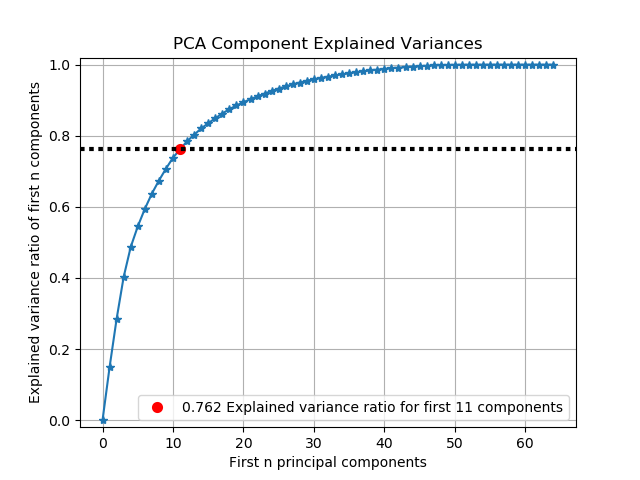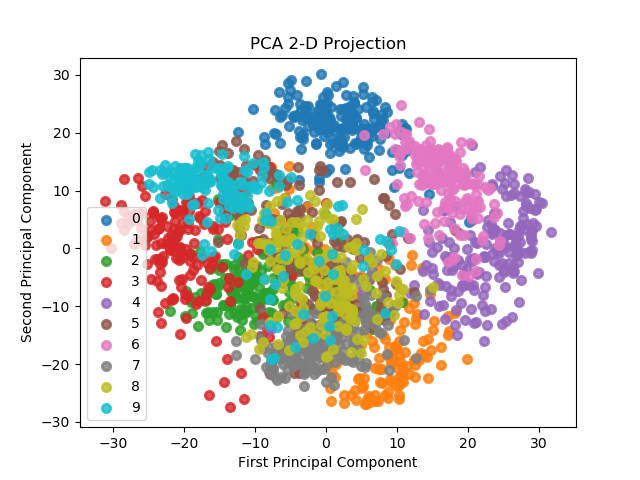# Decomposition Module (API Reference)¶

The `scikitplot.decomposition` module includes plots built specifically for scikit-learn estimators that are used for dimensionality reduction e.g. PCA. You can use your own estimators, but these plots assume specific properties shared by scikit-learn estimators. The specific requirements are documented per function.

`scikitplot.decomposition.``plot_pca_component_variance`(clf, title='PCA Component Explained Variances', target_explained_variance=0.75, ax=None, figsize=None, title_fontsize='large', text_fontsize='medium')

Plots PCA components’ explained variance ratios. (new in v0.2.2)

Parameters: clf – PCA instance that has the `explained_variance_ratio_` attribute. title (string, optional) – Title of the generated plot. Defaults to “PCA Component Explained Variances” target_explained_variance (float, optional) – Looks for the minimum number of principal components that satisfies this value and emphasizes it on the plot. Defaults to 0.75 ax (`matplotlib.axes.Axes`, optional) – The axes upon which to plot the curve. If None, the plot is drawn on a new set of axes. figsize (2-tuple, optional) – Tuple denoting figure size of the plot e.g. (6, 6). Defaults to `None`. title_fontsize (string or int, optional) – Matplotlib-style fontsizes. Use e.g. “small”, “medium”, “large” or integer-values. Defaults to “large”. text_fontsize (string or int, optional) – Matplotlib-style fontsizes. Use e.g. “small”, “medium”, “large” or integer-values. Defaults to “medium”. The axes on which the plot was drawn. ax (`matplotlib.axes.Axes`)

Example

```>>> import scikitplot as skplt
>>> pca = PCA(random_state=1)
>>> pca.fit(X)
>>> skplt.decomposition.plot_pca_component_variance(pca)
<matplotlib.axes._subplots.AxesSubplot object at 0x7fe967d64490>
>>> plt.show()
````scikitplot.decomposition.``plot_pca_2d_projection`(clf, X, y, title='PCA 2-D Projection', biplot=False, feature_labels=None, ax=None, figsize=None, cmap='Spectral', title_fontsize='large', text_fontsize='medium')

Plots the 2-dimensional projection of PCA on a given dataset.

Parameters: clf – Fitted PCA instance that can `transform` given data set into 2 dimensions. X (array-like, shape (n_samples, n_features)) – Feature set to project, where n_samples is the number of samples and n_features is the number of features. y (array-like, shape (n_samples) or (n_samples, n_features)) – Target relative to X for labeling. title (string, optional) – Title of the generated plot. Defaults to “PCA 2-D Projection” biplot (bool, optional) – If True, the function will generate and plot biplots. If false, the biplots are not generated. feature_labels (array-like, shape (n_classes), optional) – List of labels that represent each feature of X. Its index position must also be relative to the features. If `None` is given, then labels will be automatically generated for each feature. e.g. “variable1”, “variable2”, “variable3” … ax (`matplotlib.axes.Axes`, optional) – The axes upon which to plot the curve. If None, the plot is drawn on a new set of axes. figsize (2-tuple, optional) – Tuple denoting figure size of the plot e.g. (6, 6). Defaults to `None`. cmap (string or `matplotlib.colors.Colormap` instance, optional) – Colormap used for plotting the projection. View Matplotlib Colormap documentation for available options. https://matplotlib.org/users/colormaps.html title_fontsize (string or int, optional) – Matplotlib-style fontsizes. Use e.g. “small”, “medium”, “large” or integer-values. Defaults to “large”. text_fontsize (string or int, optional) – Matplotlib-style fontsizes. Use e.g. “small”, “medium”, “large” or integer-values. Defaults to “medium”. The axes on which the plot was drawn. ax (`matplotlib.axes.Axes`)

Example

```>>> import scikitplot as skplt
>>> pca = PCA(random_state=1)
>>> pca.fit(X)
>>> skplt.decomposition.plot_pca_2d_projection(pca, X, y)
<matplotlib.axes._subplots.AxesSubplot object at 0x7fe967d64490>
>>> plt.show()
```